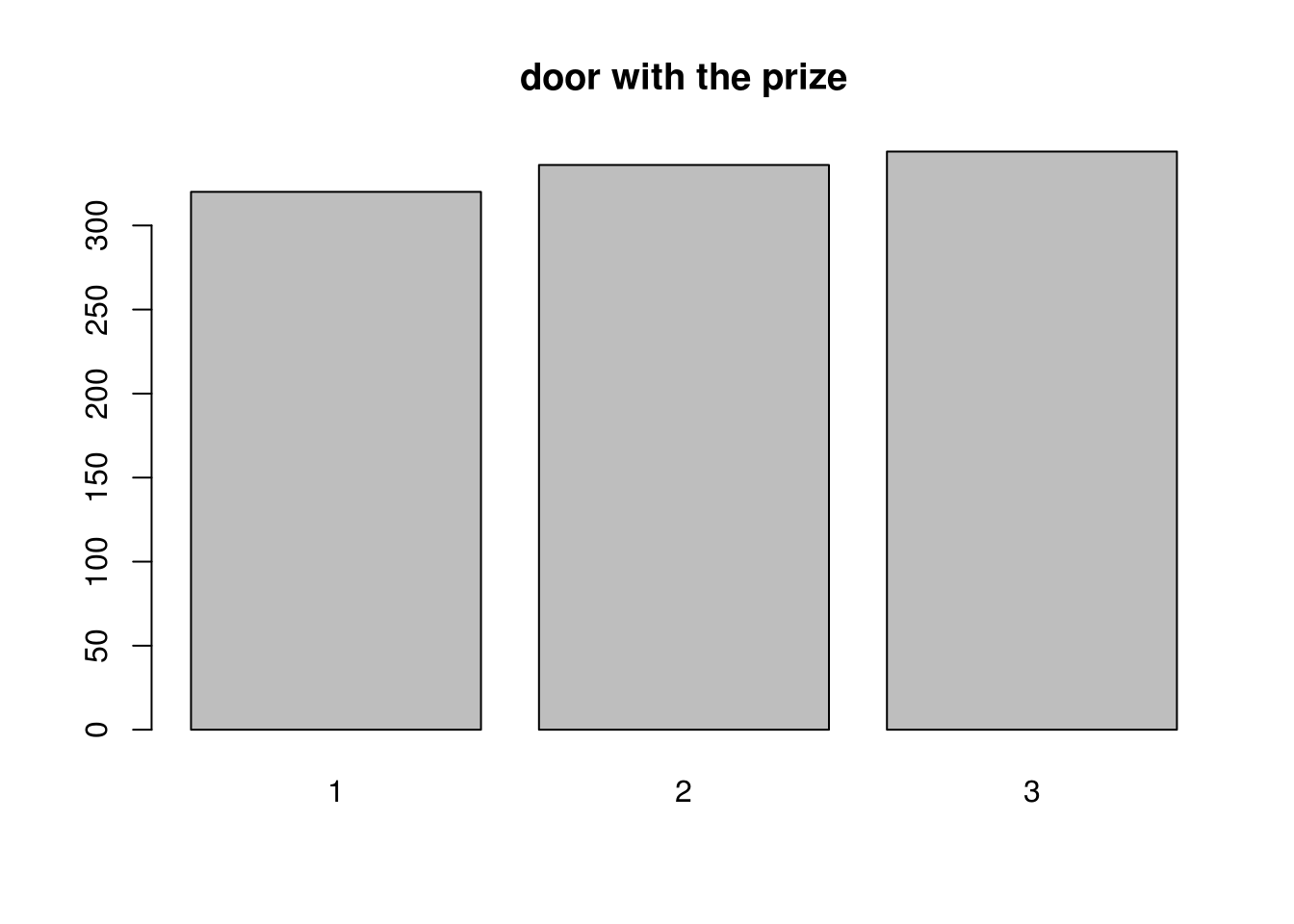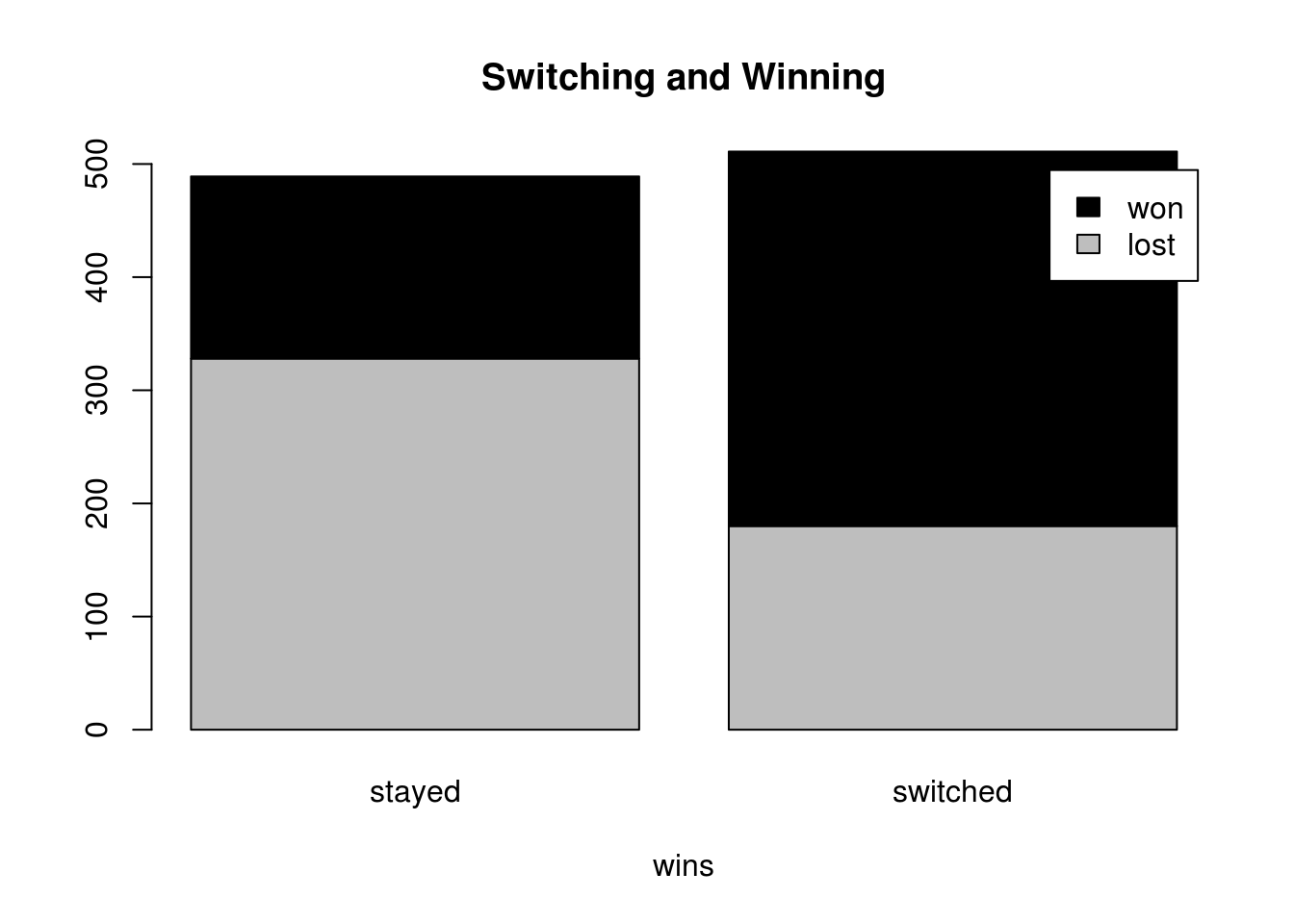## Introduction

In the classical Monty Hall problem, a game show host, named Monty Hall, presents a contestant with three (3) doors. Behind one of the doors is a valuable prize, traditionally a car, while behind the others are dud prizes, traditionally goats. A game proceeds with the contestant selecting a door and keeping the prize that lies behind it (hoping for the actual prize, not a dud). However, instead of opening the selected door, Monty opens one of the other two doors, revealing a goat, and asks if the contestant would like to switch and select the remaining door instead. The problem asks which choice has the higher probability of revealing the actual prize, or whether both options have the same probability of achieving the hoped-for outcome.

When first presented with the problem, many will naively argue that at the point where the opportunity to switch is offered there is an equal probability that the remaining doors conceal the car. It turns out, counter-intuitively for some, that the chance of getting thethe-basics car is much better if you switch.

Simulations are a nice way of exploring probability problems that sometimes defeat our intuition. This document shows how to set up a simple simulation of the Monty Hall problem that generates a data set that can be explored.

## Setting up the Simulation

First we decide how many trials to include in the simulation - let’s set up a MontyHall dataframe with 1000 trials.

trial_limit <- 1000
trial <- 1:trial_limit
MontyHall <- data.frame(trial)
str(MontyHall)
## 'data.frame':    1000 obs. of  1 variable:
##  $trial: int 1 2 3 4 5 6 7 8 9 10 ... For each trial, a door is selected (by Monty) as the winner. We populate our MontyHall dataframe with a randomly selected winning door for each. for (i in trial) { MontyHall$prizeDoor[i] <- sample(1:3,1) }

Hopefully, we see that R has done a good job of randomly distributing the prize between the three doors. A quick visual check should reassure us.Simlarly, for each trial, a door is selected by the contestant. This time, we’ll use a different way of allocating the selections.

MontyHall$firstChoice <- sample(1:3,trial_limit,replace=TRUE)  Next, we will determine if the initially selected door was the winner. Monty will keep this information from the contestant, and instead select a door to reveal as a dud. MontyHall$selectedWinner <- MontyHall$firstChoice == MontyHall$prizeDoor
for (i in trial) {
if(MontyHall$selectedWinner[i]){ MontyHall$revealedDoor[i] <-  sample(c(1:3)[- which(c(1:3) == MontyHall$firstChoice[i])],1) } else { t <- c(1:3)[-which(c(1:3)==MontyHall$firstChoice[i])];
MontyHall$revealedDoor[i] <- t[-which(t==MontyHall$prizeDoor[i])]
}
}

Once presented with a door showing a dud prize, the contestant can decide whether or not to switch. For this simulation, we will say that the contestant switches 50% of the time. The contestant’s final choice is either the door they started with, or the remaining door that was not chosen or revealed.

MontyHall$switchChoice <- sample(c(TRUE, FALSE), trial_limit, replace=TRUE) MontyHall$action[MontyHall$switchChoice] <- "switched" MontyHall$action[!MontyHall$switchChoice] <- "stayed" for (i in trial) { if (MontyHall$switchChoice[i]){
t <- c(1:3)[-which(c(1:3)==MontyHall$firstChoice[i])]; MontyHall$finalChoice[i] <- t[-which(t==MontyHall$revealedDoor[i])] } else { MontyHall$finalChoice[i] <- MontyHall$firstChoice[i] } } Finally, Monty will reveal the selected door, and we will see if the contestant won. MontyHall$winner <- MontyHall$finalChoice == MontyHall$prizeDoor
MontyHall$outcome[MontyHall$winner] <- "won"
MontyHall$outcome[!MontyHall$winner] <- "lost"

## Exploring the Simulation

We now have a data frame MontyHall that contains 1000 trials of an imagined contestant playing the game.

str(MontyHall)
## 'data.frame':    1000 obs. of  10 variables:
##  $trial : int 1 2 3 4 5 6 7 8 9 10 ... ##$ prizeDoor     : int  2 1 3 1 3 1 1 1 2 3 ...
##  $firstChoice : int 3 3 1 3 2 3 1 1 3 2 ... ##$ selectedWinner: logi  FALSE FALSE FALSE FALSE FALSE FALSE ...
##  $revealedDoor : int 1 2 2 2 1 2 3 3 1 1 ... ##$ switchChoice  : logi  FALSE TRUE TRUE FALSE TRUE TRUE ...
##  $action : chr "stayed" "switched" "switched" "stayed" ... ##$ finalChoice   : int  3 1 3 3 3 1 1 1 3 2 ...
##  $winner : logi FALSE TRUE TRUE FALSE TRUE TRUE ... ##$ outcome       : chr  "lost" "won" "won" "lost" ...

Lets take a subset of the dataset that includes only those cases where the contestant did not switch, and observe the proportion of times this was a winning strategy.

NoSwitchMonty <- subset(MontyHall, MontyHall$switchChoice == FALSE) prop.table(table(NoSwitchMonty$winner))
##
##     FALSE      TRUE
## 0.6707566 0.3292434

This looks close to the theoretical probability, which tells us that not switching will only lead to a win in one third of the cases. Conversely, switchers should prosper:

SwitchMonty <- subset(MontyHall, MontyHall$switchChoice == TRUE) prop.table(table(SwitchMonty$winner))
##
##     FALSE      TRUE
## 0.3522505 0.6477495

You might want to check and see if we really did switch fifty percent of the time, as we tried to set up in the simulation.

prop.table(table(MontyHall$switchChoice)) ## ## FALSE TRUE ## 0.489 0.511 Based on the law of total probability, switching half of the time we should expect to win half of the time. You might want to check and see if we really did switch fifty percent of the time, as we tried to set up in the simulation. prop.table(table(MontyHall$winner))
##
## FALSE  TRUE
## 0.508 0.492

Of course, most of these wins (two thirds) came from those trials where the contestant switched away from their original choice.

t <- table(MontyHall$outcome, MontyHall$action)
barplot(t, main="Switching and Winning",
xlab="wins", col=c("grey","black"),
legend = rownames(t))